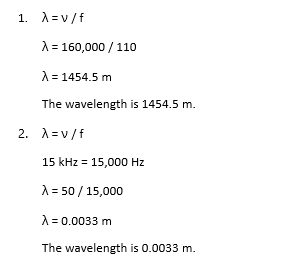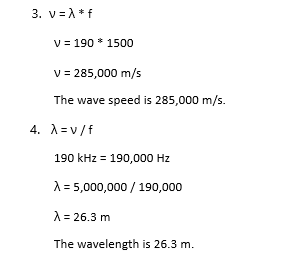# How to Calculate Wavelength

Lesson Transcript
Instructor
Thomas Zesiger

Thomas has taught electronics and communications engineering, math, and physics and has a master's degree in electrical engineering.

Expert Contributor
Dawn Mills

Dawn has taught chemistry and forensic courses at the college level for 9 years. She has a PhD in Chemistry and is an author of peer reviewed publications in chemistry.

Discover practical examples of waves and the significance of wavelength. Learn the relationship of wavelength, frequency, and wave speed, as well as how to calculate wavelength based on these parameters. Updated: 10/31/2020

## Wavelength

Ripples in water, sound traveling in air, and coordinated vibrations of objects are examples of waves you have probably encountered in your life. A good way to visualize a wave is to insert the end of a pencil into a container of still water. The surface of the water is disturbed, producing ripples, or waves.

Electromagnetic waves are special waves, such as light, radio waves, microwaves, and x-rays, that do not require a medium for propagation. We cannot see or hear these waves, but they exist in nature and in many of the products we use every day.

Regardless of the kind, every wave has a wavelength. Wavelength is the distance between two successive like points on a wave. Some examples are the distance between two adjacent peaks or two adjacent valleys. A peak is the highest point of a wave and a valley is the lowest point of a wave. Stated another way, wavelength is the time required to complete one full cycle of the wave.

Wavelength depends on two other important parameters:

#### 1. Wave Speed

The rate at which the wave moves through the medium of propagation. Wave speed is dependent upon the medium of propagation. For example, water ripples travel through the water. Electromagnetic waves usually travel through the air, as do sound waves. Vibrations on a piano string travel through the string. The wave speed is different for all of these because the medium in which the wave propagates is different.

#### 2. Frequency

The number of wave cycles passing a point per unit time. Stated another way, it is the number of oscillations per second in the wave. A higher frequency means a shorter wavelength, and a shorter wavelength means a higher frequency. This leads us to the relationship between wave speed, frequency, and wavelength.

An error occurred trying to load this video.

Try refreshing the page, or contact customer support.

Coming up next: Incident Ray: Definition & Overview

### You're on a roll. Keep up the good work!

Replay
Your next lesson will play in 10 seconds
• 0:04 Wavelength
• 1:52 Calculating Wavelength
• 2:34 Examples
• 3:25 Lesson Summary
Save Save

Want to watch this again later?

Log in or sign up to add this lesson to a Custom Course.

Timeline
Autoplay
Autoplay
Speed Speed

## Calculating Wavelength

The speed of the wave equals the number of cycles passing a point each second multiplied by the cycle length.

Mathematically stated: wave speed = cycles per second x cycle length

To unlock this lesson you must be a Study.com Member.
Create your account

Additional Activities

## Calculating Wavelength

Wavelength exists in different products and applications we use in our everyday lives such as microwaves, the radio, and how we see and interpret colors. The problems below will assist you in calculating the wavelength from different scenarios and sets of information. Wavelength can be determined based on its relationship with frequency and wave speed. The solutions are provided in order to further understand the calculations step by step.

### Practice Problems

1. Calculate the wavelength of a sound wave that has a frequency of 110 Hz and a wave speed of 160,000 m/s.

2. What is the wavelength of an unidentified wave that has a wave speed of 50 m/s and a frequency of 15 kHz?

3. The wavelength of a specific note in a song played on the radio was 190 m. The frequency was determined to be 1500 Hz. What is the wave speed of this note?

4. Determine the wavelength of a wave based on the parameters provided. The wave has a frequency of 190 kHz and a wave speed of 5,000,000 m/s.

### Solutions### Register to view this lesson

Are you a student or a teacher?

### Unlock Your Education

#### See for yourself why 30 million people use Study.com

##### Become a Study.com member and start learning now.
Back
What teachers are saying about Study.com
Create an account to start this course today
Used by over 30 million students worldwide